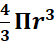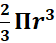# RD Sharma Class 9 and Chapter Surface Area And Volume Of A Sphere Maths Solutions

## Maths Class 9 and Chapter Surface Area And Volume Of A Sphere RD Sharma SolutionsFilter
Filters :
CLASSES
•
•
•
•
•
•
•
CHAPTERS
•
• 15 More

### RD SHARMA Class 9 | SURFACE AREA AND VOLUME OF A SPHERE

A measuring jar of internal diameter 10cm is partially filled with water. Four equal spherical balls of diameter 2cm each are dropped in it and they sink down in water completely. What will be the change in the level of water in the jar?A sphere is a figure that takes round shape and is not same as a circle. The NCERT textbook definition of a sphere goes as, “a sphere is a three-dimensional figure (solid figure), which is made up of all points in the space, which lie at a constant distance called the radius, from a fixed point called the centre of the sphere”.

The sphere is a round three-dimensional figure that is formed by a set of points that lie at a constant radius from a fixed centre point. So, a sphere is not a circle. Unlike a circle, a sphere cannot be drawn on a paper.  Let us take an example to understand the difference between these two figures. A ball is an example for a sphere and example for a circle would be a wheel or a circular plate. Now take the fruit orange for an example. It takes the shape of a sphere. Now cut a slice from the middle of the orange thus dividing it into two parts. Now, this slice takes the shape of a circle and this cut circular slice can divide the spherical orange into two parts. Now when a sphere is divided into two parts, one can notice two ‘hemispheres’ being formed. So, this unit also talks about the surface area and volume of hemisphere along with the sphere.

Looking at the formulae derived for calculating the area and volume of spheres and hemispheres, covered under this chapter, RD Sharma Class 9 Maths Chapter 21 Surface Area and Volume of a Sphere:

The Surface Area of a Sphere = 4 π r2

The Curved Surface Area of a Hemisphere = 2πr2

The Total Surface Area of a Hemisphere = 3πr2

The Volume of a Sphere =The Volume of a Hemisphere =Here, ‘r’ is the radius of the sphere.

Knowing to apply these formulae tactically while solving the problems is important. These tactics and concepts are well covered in RD Sharma’s textbook. RD Sharma’s books are the most trusted ones in the CBSE Board Maths textbook category. This is because of the reason that RD Sharma’s textbooks detail all the concepts in an easily comprehensible method. All the maths problems are worked out in a step-by-step way. RD Sharma’s books also offer a lot of solved examples that serve very helpful for the students to understand the different approaches involved in solving problems. Not only that, one can also find ample problems provided in the form of exercises in the textbook. Working out these problems have proved to be helpful not just to face the school exams, but also ace through the other tough competitive entrance examinations like the IIT JEE, BITSAT, VITEE, etc.

As much owing a good textbook is important, getting hold of a related solution guide for the textbook, especially for a concept-based subject like Maths, stands equally important. In this line comes our innovative technology-based platform, the Doubtnut.  Doubtnut is an online tutorial platform that provides video solutions and PDF solutions for different Maths textbooks. Coming to the current chapter that covers all about the sphere in RD Sharma’s textbook, the exercise-wise solutions are found on Doubtnut as RD Sharma Class 9 Solutions Chapter 21 Surface Area and Volume of a Sphere.

## Why Doubtnut for this chapter, Surface Area and Volume of a Sphere?

Doubtnut being one of the very few tutorials platforms that provides textbook solutions in the form of videos has hence managed to grab the attention of thousands of students across India. All the video solutions are curated by a team of brilliant subject matter experts. Each and every exercise problem from this chapter is presented in the form of video tutorials and this makes the students grasp all the geometrical aspects thoroughly. Not just the RD Sharma books, one can find the video solutions on Doubtnut for other maths textbooks too. The NCERT maths solutions are also available on Doubtnut, and these are available in the form of PDFs too. Apart from these, in case of any doubts from this chapter need not be accumulated to get them answered later, anymore. All the doubts can be clarified within a short period of time with the help of Doubtnut. Doubtnut users are welcome to get any of their doubts or questions, by simply posting pictures of the questions from the textbook either on the app or the website.

## Topics and Subtopics of RD Sharma Class 9 Maths Chapter 21 Surface Area and Volume of a Sphere

Knowing the topics and subtopics in a unit beforehand given a clear picture of what the entire chapter is about. Take any chapter from any subject, let alone Maths, it is essential to be aware of the topics and subtopics that the chapter consists of. This knowledge helps them to better plan the time allotment and the study timetable well in advance.

Listing the topics and subtopics of RD Sharma class 9 maths chapter 21 Surface Area and Volume of a Sphere:

### Introduction to a sphere

The definition and introduction into the circle-like figure, the sphere, is covered. The related terms are also included here. The related figure called the hemisphere is also defined here and the one can understand clearly how a hemisphere is formed out of a sphere under this topic.

### The surface area of a sphere and hemisphere

The derivation of the surface area of a sphere and also the hemisphere is done under this topic. All the related terms such as the radius of the sphere that is inculcated in the formula are elaborated under this topic. One can find several exercise problems being covered under this topic.

### The volume of a sphere and hemisphere

The derivation of the formula for calculating the volumes of a sphere and a hemisphere are covered under this topic. Again, one can find a lot of exercise problems in the textbook that are based on these volume formulae.

## Briefing out a few advantages of our platform Doubtnut for the students

Doubtnut has raced on to become one of the premium online learning platforms. One can find video solutions for all the textbook problems which are worked out methodically. This method of learning helps the students to understand the concepts better as learning through videos serves as the premium learning methodology since the learners would be able to retain the learnt lessons better. The language used to explain the concepts is very simple so that it can be understood easily by the students, thus making it more student-friendly. The simple language usage and the immersive learning technology, together that help users learn the lessons effortlessly, have made Doubtnut a highly trusted online tutorial medium in recent times. As already mentioned, Doubtnut makes it effortlessly easy for the learners to get their questions from the chapter answered and any doubts cleared in the right way. This is an important factor that would help the students in eliminating unnecessary investment of time on the concepts and methods that are not clear to them. Thus, Doubtnut serves as a great platform that encourages swift learning. Apart from all these, Doubtnut offers all these video solutions and materials for free of cost. This makes it very much approachable and highly accessible for students from all over the country irrespective of their financial backgrounds. Doubtnut thus enables the students who don’t have the access to attend any kind of tutorial classes or cannot afford to buy any solution materials to study by self and thus face the examinations with great confidence.

Share this on :-